LearnFast.Ninja

# Calculate mean in Excel 2010

This tutorial will show you how to calculate mean in Excel 2010. All you need is to use the average function.

Step 1: Create cell for mean.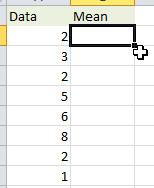Step 2: Click Formulas then Insert Function.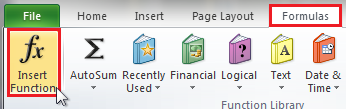Step 3: Click AVERAGE then click OK.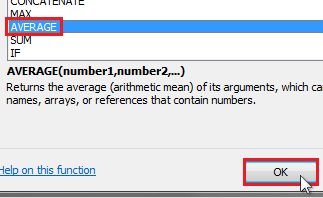Step 4: Select the data range.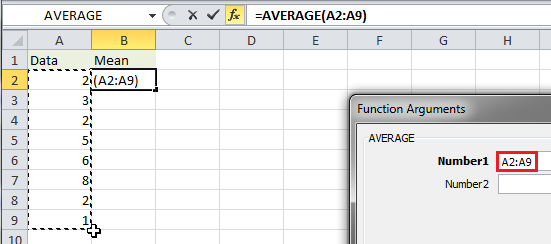Result: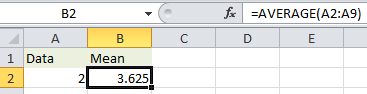Tags

excel